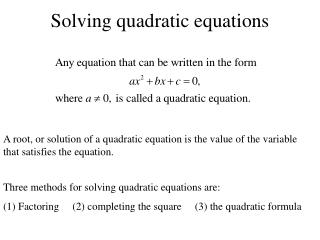DownloadDownload PresentationTélécharger la présentation- - - - - - - - - - - - - - - - - - - - - - - - - - - E N D - - - - - - - - - - - - - - - - - - - - - - - - - - -
##### Presentation Transcript

1. Solving quadratic equations A root, or solution of a quadratic equation is the value of the variable that satisfies the equation. Three methods for solving quadratic equations are: (1) Factoring (2) completing the square (3) the quadratic formula

2. Factoring Whenever the product of two factors is zero, at least one of the factors must be zero.

3. Ex. 1. Solve

4. Completing the square • Divide both sides by the coefficient of so that will have a coefficient of 1 • Subtract the constant term from both sides • Add the square of one half the coefficient of x to both sides • Take the square root of both sides and solve for x Ex. 2. Solve:

5. The quadratic formula The roots of the quadratic equation: Are given by: Ex. 3. Solve

6. Discriminant: the quantity that appears beneath the radical sign in the quadratic formula tells you whether the roots of a quadratic equation are real or imaginary. There are two conjugate imaginary roots. There is one real root (double root) There are two different real roots

7. Choosing a Method of Solution Although you can use the quadratic formula to solve any equation, it is often much easier to factor or complete the square. The list below suggests when to use which method.

8. If an equation contains variables on both sides or variables in the denominator, then you must carefully organize your method for solving in order not to lose a root or gain a root. It is possible to lose a root by dividing both sides of an equation by a common factor.

9. Two ways to avoid losing a root are shown below. Both methods are correct. Method 1 Method 2 If there is a factor common to both sides of the equation, remember to include as roots all values that make this factor zero. Bring all terms to one side of the equation and then solve.

10. Gaining a root Squaring both sides or multiplying by an expression may give you an extraneous root which satisfies the transformed equation but not the original one. Multiply each side by the LCD

11. Check: Undefined, so x = 2 is not a solution.